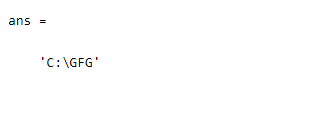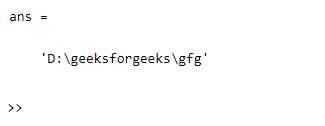Set environment variable in MATLAB

• Last Updated : 04 Aug, 2021

Setting environment variables with the help of setenv() function. The setenv() function is used to set the specified value of an operating system environment variable. Here the environment variable “name” will be replaced with “value”, where “name” and “value” is its parameters.

Syntax

setenv(name, value)

Parameters: This function accepts two parameters, which are illustrated below:

• name : This is the specified existing environmental variable.
• values: This is the specified variable that replaces the “name” variable.

Return Value: It returns the replaced variable name.

Example 1

Matlab

 % MATLAB code for environment variable% GFG has been created and later replaced % with "C:\GFG" using the setenv() functionsetenv('GFG','C:\GFG');  % Getting the current variable namegetenv('GFG')

Output:Example 2

Matlab

 % MATLAB code for setenv() function to % set the value D:\geeksforgeeks\gfg over % the variable "a"setenv('a','D:\geeksforgeeks\gfg');  % Getting the current variable namegetenv('a')

Output:My Personal Notes arrow_drop_up